# Write an equation for a rational function m with: Use the smallest powers possible to meet...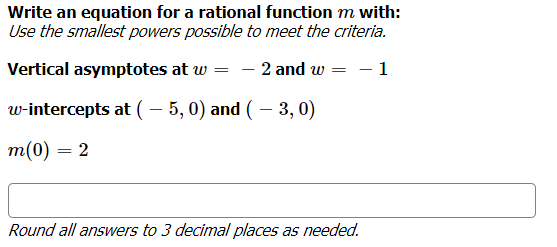Write an equation for a rational function m with: Use the smallest powers possible to meet the criteria. Vertical asymptotes at w 2 and w = -1 w-intercepts at ( -5,0) and (-3,0) m(0) = 2 Round all answers to 3 decimal places as needed.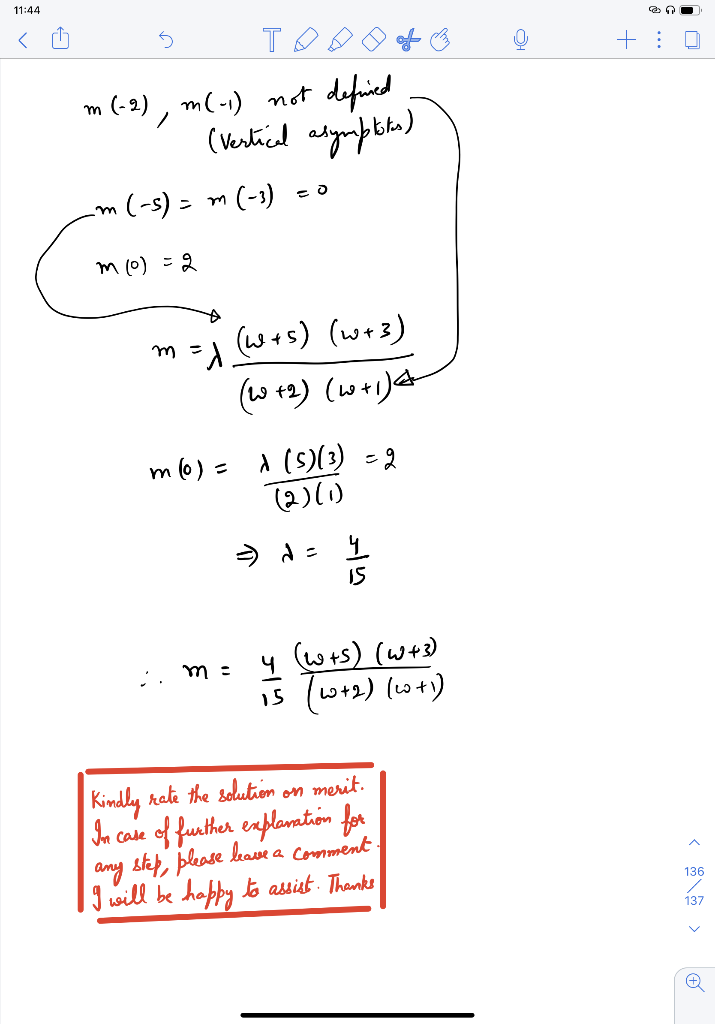##### Add Answer of: Write an equation for a rational function m with: Use the smallest powers possible to meet...
Similar Homework Help Questions
• ### Write an equation for the function graphed below. Use the smallest powers possible to meet the...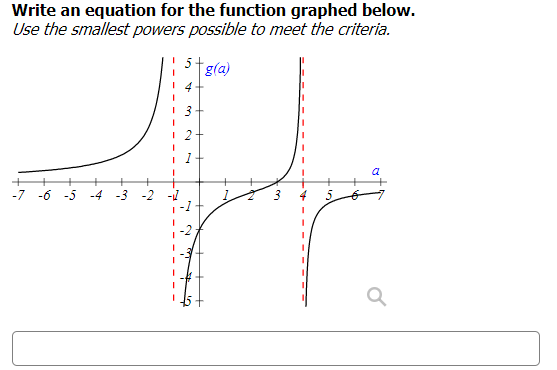Write an equation for the function graphed below. Use the smallest powers possible to meet the criteria. stgla) 4 3 a + -7 -6 -5 -4 -3 -2 3 2

• ### Write an equation for the function graphed below. Use the smallest powers possible to meet the...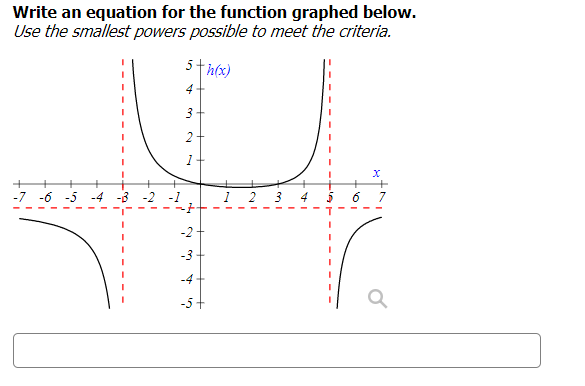Write an equation for the function graphed below. Use the smallest powers possible to meet the criteria. 5th(x) 4+ 3+ 2+ 1 X -7 -6 -5 -4 -8 -2 3 4 6 To 1 -2+ 3 -4 -5

• ### equation of rational function!!!!

Find an equation of a rational function (f) which satisfies thefollowing:x int: (-5,0)hole (1,4)vertical asymptotes x = 3 and x = -2

• ### Write an equation for a rational function with: Vertical asymptotes at x = -3 and x...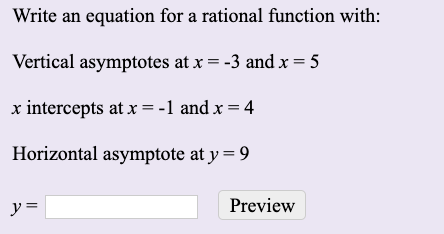Write an equation for a rational function with: Vertical asymptotes at x = -3 and x = 5 x intercepts at x = -1 and x = 4 Horizontal asymptote at y=9 y = Preview

• ### The graph of a rational function fis shown below. Assume that all asymptotes and intercepts are...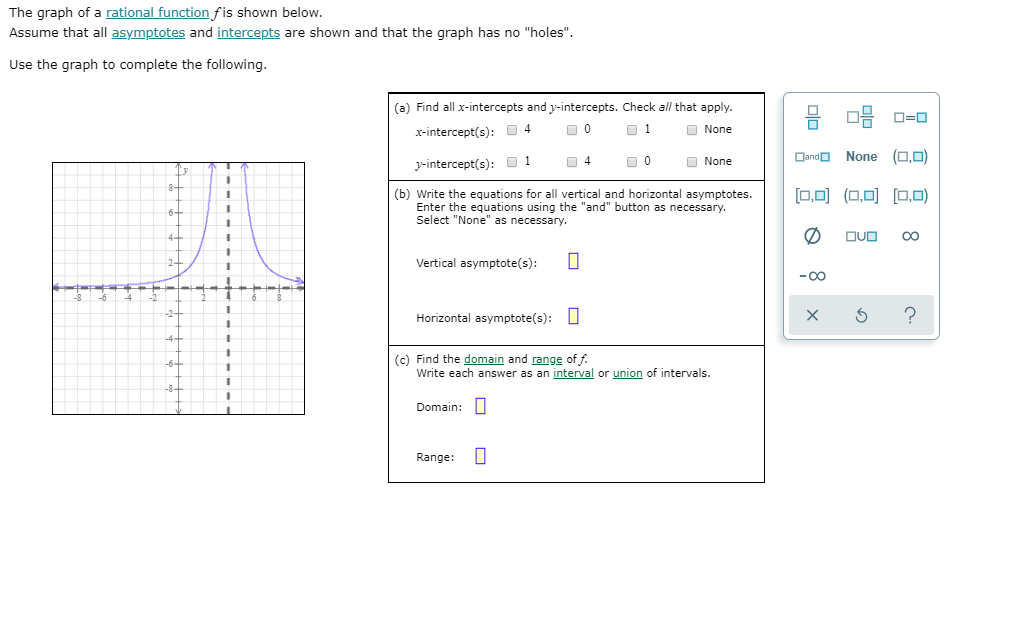The graph of a rational function fis shown below. Assume that all asymptotes and intercepts are shown and that the graph has no "holes". Use the graph to complete the following. (a) Find all x-intercepts and y-intercepts. Check all that apply. X-intercept(s): 4 00 01 None . : O=D y-intercept(s): 01 04 00 None Dando None (0,0) HHH [0,0] (0,0] [0,0) (b) Write the equations for all vertical and horizontal asymptotes. Enter the equations using the "and" button as necessary....

• ### Please tell me which options I need to select and what I have to type in. Thank you! 3-3x For the given rational function f(x)- x- find the following (A) Find the intercepts for the graph. (B) Determi...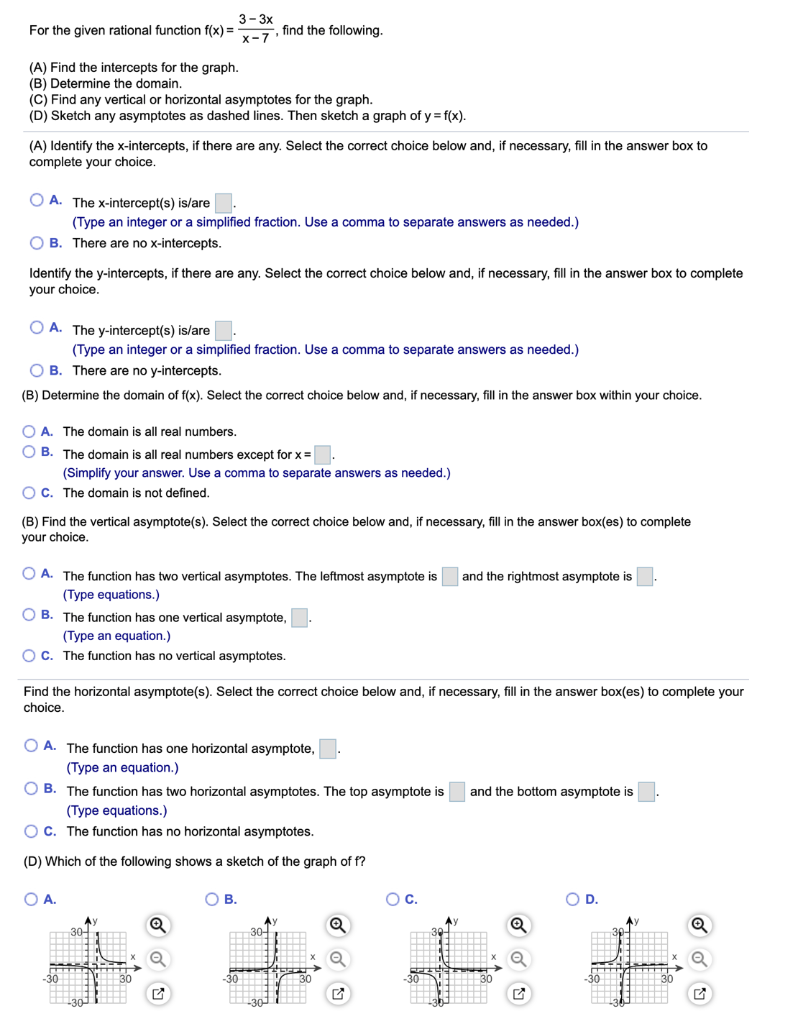Please tell me which options I need to select and what I have to type in. Thank you! 3-3x For the given rational function f(x)- x- find the following (A) Find the intercepts for the graph. (B) Determine the domain. (C) Find any vertical or horizontal asymptotes for the graph (D) Sketch any asymptotes as dashed lines. Then sketch a graph of y f(x) (A) Identify the x-intercepts, if there are any. Select the correct choice below and, if necessary,...

• ### Is it possible to find a rational function that has x-intercepts (-2,0) and (2,0), but has vertical asymptote x=1 and horizontal asymptote of y=0

Is it possible to find a rational function that has x-intercepts (-2,0) and (2,0), but has vertical asymptote x=1 and horizontal asymptote of y=0? The horizontal asymptote and the x-intercepts parts stump me. If you can't reach y=0, then how can you get the x-intercepts? And I don't know how to start finding a rational function that has asymptotes x=0 and y=x. It seems like I only have enough space to place a function in Q-2, Q-4.Well, you can reach...

• ### Use the following information to construct a suitable rational function f(x)

Use the following information to construct a suitable rational function f(x).* There is a vertical asymptote at x=2, and a horizontal asymptote at y=3.* The graph of the function must go through the points (-4/3,0) and (0,-2)

• ### Analyzing the graph of a rational function

R(X)=6___X^2-X-6Please show all these steps so I can figure out how to do it!!1. Factor and the domain of the rational function.2. Write in lowest terms.3. Locate intercepts of the graph, determine behavior of the graph near each x-intercept. Then plot them and indicate the behavior of the graph near it.4. Locate vertical asymptotes and graph.5. Locate horizontal or oblique asymptote.6.Use the zeros of the numerator and denominator of R to divide the x-axis into intervals. Determine if the graph...

• ### Use the following function to answer parts a through a. f(x) = x2 + 4x2 -...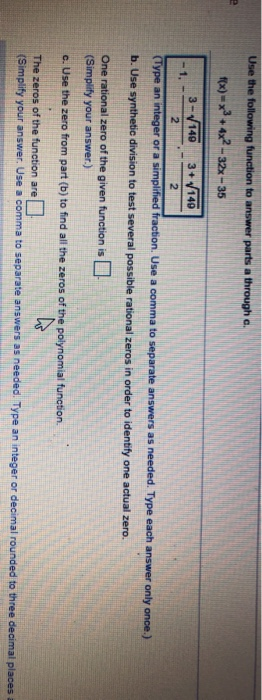Use the following function to answer parts a through a. f(x) = x2 + 4x2 - - 32x - 35 3-140 3+ 140 1. 2 2 (Type an integer or a simplified fraction. Use a comma to separate answers as needed. Type each answer only once.) b. Use synthetic division to test several possible rational zeros in order to identify one actual zero. One rational zero of the given function is (Simplify your answer.) c. Use the zero from part...

Free Homework App